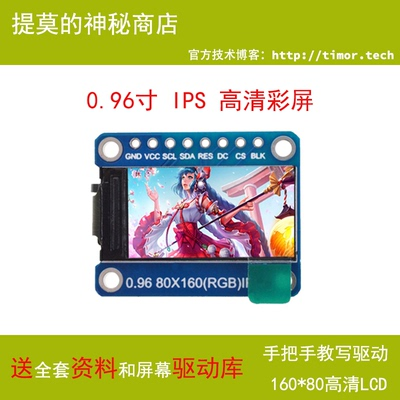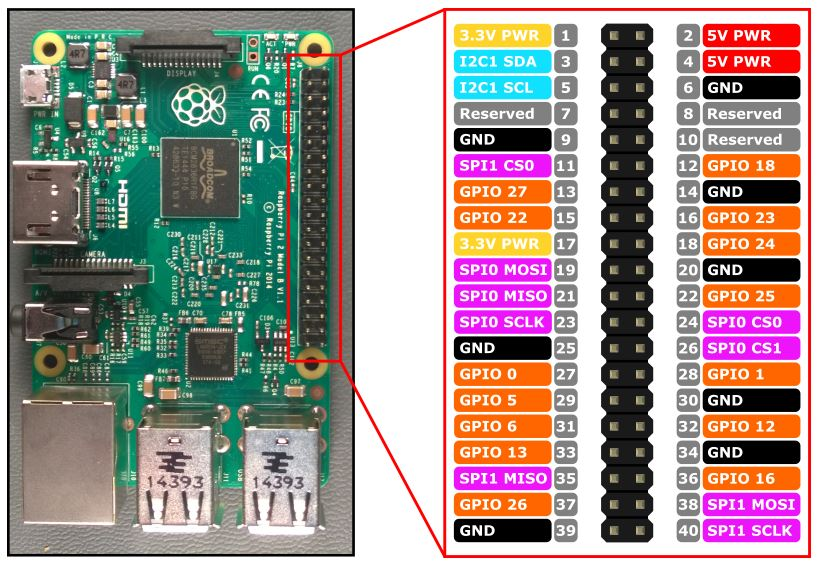# 树莓派使用 Python 编写/移植 ST7735S 0.96 寸彩色 LCD 屏幕驱动（SPI 通信）## 打开硬件 SPI

``````\$ ls /dev/spi*
``````

## 硬件接线## 驱动移植

``````#include<reg51.h>
#include<absacc.h>
#include<intrins.h>
#include<string.h>
#define uchar unsigned char
#define uint unsigned int

//如果您使用的单片机不是STC12系列(如STC89C52)请屏蔽此宏定义
#define MCU_STC12
#ifdef MCU_STC12
sfr P3M1  = 0xB1;
sfr P3M0  = 0xB2;
#endif

//---------------------------液晶屏接线说明-------------------------------------//
//接线前请参考液晶屏说明书第10页引脚定义
sbit bl        =P1^5; //接模块BL引脚，背光可以采用IO控制或者PWM控制，也可以直接接到高电平常亮
sbit scl       =P1^0; //接模块CLK引脚,接裸屏Pin9_SCL
sbit sda       =P1^1; //接模块DIN/MOSI引脚，接裸屏Pin8_SDA
sbit rs        =P1^3; //接模块D/C引脚，接裸屏Pin7_A0
sbit cs        =P1^4; //接模块CE引脚，接裸屏Pin12_CS
sbit reset     =P1^2; //接模块RST引脚，接裸屏Pin6_RES
//---------------------------End of液晶屏接线---------------------------------//

//定义常用颜色
#define RED     0xf800
#define GREEN   0x07e0
#define BLUE    0x001f
#define WHITE   0xffff
#define BLACK   0x0000
#define YELLOW  0xFFE0
#define GRAY0   0xEF7D
#define GRAY1   0x8410
#define GRAY2   0x4208

void delay_ms(uint time)
{
uint i, j;
for(i = 0; i < time; i++)
for(j = 0; j < 250; j++);
}

//向SPI总线传输一个8位数据
void SPI_WriteData(uchar Data)
{
unsigned char i=0;
for(i=8;i>0;i--)
{
if(Data&0x80)
sda=1;
else
sda=0;
scl=0;
scl=1;
Data<<=1;
}
}

//向液晶屏写一个8位指令
void Lcd_WriteIndex(uchar Data)
{
cs=0;
rs=0;
SPI_WriteData(Data);
cs=1;
}

//向液晶屏写一个8位数据
void Lcd_WriteData(uchar Data)
{
unsigned char i=0;
cs=0;
rs=1;
SPI_WriteData(Data);
cs=1;
}

//向液晶屏写一个16位数据
void LCD_WriteData_16Bit(unsigned int Data)
{
unsigned char i=0;
cs=0;
rs=1;
SPI_WriteData(Data>>8); //写入高8位数据
SPI_WriteData(Data); //写入低8位数据
cs=1;
}

void Reset()
{
reset=0;
delay_ms(100);
reset=1;
delay_ms(100);
}

void Lcd_initial()
{
Reset(); //Reset before LCD Init.

Lcd_WriteIndex(0x11); // Sleep exit
delay_ms (120);

Lcd_WriteIndex(0x21);
Lcd_WriteIndex(0xB1);
Lcd_WriteData(0x05);
Lcd_WriteData(0x3A);
Lcd_WriteData(0x3A);
Lcd_WriteIndex(0xB2);
Lcd_WriteData(0x05);
Lcd_WriteData(0x3A);
Lcd_WriteData(0x3A);
Lcd_WriteIndex(0xB3);
Lcd_WriteData(0x05);
Lcd_WriteData(0x3A);
Lcd_WriteData(0x3A);
Lcd_WriteData(0x05);
Lcd_WriteData(0x3A);
Lcd_WriteData(0x3A);
Lcd_WriteIndex(0xB4);
Lcd_WriteData(0x03);
Lcd_WriteIndex(0xC0);
Lcd_WriteData(0x62);
Lcd_WriteData(0x02);
Lcd_WriteData(0x04);
Lcd_WriteIndex(0xC1);
Lcd_WriteData(0xC0);
Lcd_WriteIndex(0xC2);
Lcd_WriteData(0x0D);
Lcd_WriteData(0x00);
Lcd_WriteIndex(0xC3);
Lcd_WriteData(0x8D);
Lcd_WriteData(0x6A);
Lcd_WriteIndex(0xC4);
Lcd_WriteData(0x8D);
Lcd_WriteData(0xEE);
Lcd_WriteIndex(0xC5);
Lcd_WriteData(0x0E);
Lcd_WriteIndex(0xE0);
Lcd_WriteData(0x10);
Lcd_WriteData(0x0E);
Lcd_WriteData(0x02);
Lcd_WriteData(0x03);
Lcd_WriteData(0x0E);
Lcd_WriteData(0x07);
Lcd_WriteData(0x02);
Lcd_WriteData(0x07);
Lcd_WriteData(0x0A);
Lcd_WriteData(0x12);
Lcd_WriteData(0x27);
Lcd_WriteData(0x37);
Lcd_WriteData(0x00);
Lcd_WriteData(0x0D);
Lcd_WriteData(0x0E);
Lcd_WriteData(0x10);
Lcd_WriteIndex(0xE1);
Lcd_WriteData(0x10);
Lcd_WriteData(0x0E);
Lcd_WriteData(0x03);
Lcd_WriteData(0x03);
Lcd_WriteData(0x0F);
Lcd_WriteData(0x06);
Lcd_WriteData(0x02);
Lcd_WriteData(0x08);
Lcd_WriteData(0x0A);
Lcd_WriteData(0x13);
Lcd_WriteData(0x26);
Lcd_WriteData(0x36);
Lcd_WriteData(0x00);
Lcd_WriteData(0x0D);
Lcd_WriteData(0x0E);
Lcd_WriteData(0x10);
Lcd_WriteIndex(0x3A);
Lcd_WriteData(0x05);
Lcd_WriteIndex(0x36);
Lcd_WriteData(0xC8);
Lcd_WriteIndex(0x29);
}

/*************************************************

*************************************************/
void Lcd_SetRegion(unsigned int x_start,unsigned int y_start,unsigned int x_end,unsigned int y_end)
{
Lcd_WriteIndex(0x2a);
Lcd_WriteData(0x00);
Lcd_WriteData(x_start+0x1A);
Lcd_WriteData(0x00);
Lcd_WriteData(x_end+0x1A);

Lcd_WriteIndex(0x2b);
Lcd_WriteData(0x00);
Lcd_WriteData(y_start+1);
Lcd_WriteData(0x00);
Lcd_WriteData(y_end+1);
Lcd_WriteIndex(0x2c);
}

void Lcd_fill_color(int color)
{
uchar i,j;
Lcd_SetRegion(0,0,80-1,160-1);
for (i=0;i<160;i++)
for (j=0;j<80;j++)
LCD_WriteData_16Bit(color);
}

void main(void)
{
Lcd_initial();
Lcd_fill_color(YELLOW);
}
``````

## 移植到 树莓派

``````# -*- coding: UTF-8 -*-

import time
from luma.core.interface.serial import spi
from luma.core.render import canvas
from PIL import Image, ImageDraw, ImageFont

serial = spi(port=0, device=0, bus_speed_hz=16000000)

def delay_ms(ms):
time.sleep(ms * 0.001)

def lcd_writeCmd(cmd):
serial.command(cmd)

def lcd_writeData(data):
serial.data([data])

def lcd_reset():
serial._gpio.output(serial._RST, serial._gpio.LOW)
delay_ms(100)
serial._gpio.output(serial._RST, serial._gpio.HIGH)

def lcd_init():
lcd_reset()
lcd_writeCmd(0x11)
delay_ms(120)
lcd_writeCmd(0x21)
lcd_writeCmd(0xB1)
lcd_writeData(0x05)
lcd_writeData(0x3A)
lcd_writeData(0x3A)
lcd_writeCmd(0xB2)
lcd_writeData(0x05)
lcd_writeData(0x3A)
lcd_writeData(0x3A)
lcd_writeCmd(0xB3)
lcd_writeData(0x05)
lcd_writeData(0x3A)
lcd_writeData(0x3A)
lcd_writeData(0x05)
lcd_writeData(0x3A)
lcd_writeData(0x3A)
lcd_writeCmd(0xB4)
lcd_writeData(0x03)
lcd_writeCmd(0xC0)
lcd_writeData(0x62)
lcd_writeData(0x02)
lcd_writeData(0x04)
lcd_writeCmd(0xC1)
lcd_writeData(0xC0)
lcd_writeCmd(0xC2)
lcd_writeData(0x0D)
lcd_writeData(0x00)
lcd_writeCmd(0xC3)
lcd_writeData(0x8D)
lcd_writeData(0x6A)
lcd_writeCmd(0xC4)
lcd_writeData(0x8D)
lcd_writeData(0xEE)
lcd_writeCmd(0xC5)
lcd_writeData(0x0E)
lcd_writeCmd(0xE0)
lcd_writeData(0x10)
lcd_writeData(0x0E)
lcd_writeData(0x02)
lcd_writeData(0x03)
lcd_writeData(0x0E)
lcd_writeData(0x07)
lcd_writeData(0x02)
lcd_writeData(0x07)
lcd_writeData(0x0A)
lcd_writeData(0x12)
lcd_writeData(0x27)
lcd_writeData(0x37)
lcd_writeData(0x00)
lcd_writeData(0x0D)
lcd_writeData(0x0E)
lcd_writeData(0x10)
lcd_writeCmd(0xE1)
lcd_writeData(0x10)
lcd_writeData(0x0E)
lcd_writeData(0x03)
lcd_writeData(0x03)
lcd_writeData(0x0F)
lcd_writeData(0x06)
lcd_writeData(0x02)
lcd_writeData(0x08)
lcd_writeData(0x0A)
lcd_writeData(0x13)
lcd_writeData(0x26)
lcd_writeData(0x36)
lcd_writeData(0x00)
lcd_writeData(0x0D)
lcd_writeData(0x0E)
lcd_writeData(0x10)
lcd_writeCmd(0x3A)
lcd_writeData(0x05)
lcd_writeCmd(0x36)
lcd_writeData(0xC8)
lcd_writeCmd(0x29)

def lcd_setRegion(x1, y1, x2, y2):
lcd_writeCmd(0x2a)
lcd_writeData(0x00)
lcd_writeData(x1 + 0x1A)
lcd_writeData(0x00)
lcd_writeData(x2 + 0x1A)
lcd_writeCmd(0x2b)
lcd_writeData(0x00)
lcd_writeData(y1 + 1)
lcd_writeData(0x00)
lcd_writeData(y2 + 1)
lcd_writeCmd(0x2c)

def lcd_fill(color1, color2):
lcd_setRegion(0, 0, 79, 159)
for i in range(1,161):
for j in range(1,81):
lcd_writeData(color1)
lcd_writeData(color2)

lcd_init()
lcd_fill(0xf8, 0x00)
lcd_fill(0xff, 0xe0)
serial.cleanup()
``````